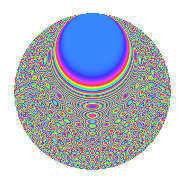# Properties

 Label 1890.2.csLevel 1890 Weight 2 Character orbit cs Rep. character $$\chi_{1890}(41,\cdot)$$ Character field $$\Q(\zeta_{18})$$ Dimension 576 Sturm bound 864

# Related objects

## Defining parameters

 Level: $$N$$ = $$1890 = 2 \cdot 3^{3} \cdot 5 \cdot 7$$ Weight: $$k$$ = $$2$$ Character orbit: $$[\chi]$$ = 1890.cs (of order $$18$$ and degree $$6$$) Character conductor: $$\operatorname{cond}(\chi)$$ = $$189$$ Character field: $$\Q(\zeta_{18})$$ Sturm bound: $$864$$

## Dimensions

The following table gives the dimensions of various subspaces of $$M_{2}(1890, [\chi])$$.

Total New Old
Modular forms 2640 576 2064
Cusp forms 2544 576 1968
Eisenstein series 96 0 96

## Trace form

 $$576q + 12q^{9} + O(q^{10})$$ $$576q + 12q^{9} - 12q^{11} - 6q^{14} + 24q^{21} + 144q^{23} - 12q^{29} + 12q^{36} + 12q^{39} - 18q^{49} + 72q^{51} - 6q^{56} + 48q^{57} + 72q^{63} + 288q^{64} + 24q^{65} - 36q^{70} + 144q^{71} + 72q^{74} + 12q^{77} + 96q^{78} + 72q^{79} + 12q^{81} - 12q^{84} + 72q^{85} + 96q^{86} + 36q^{91} - 72q^{92} + 96q^{93} - 72q^{98} - 216q^{99} + O(q^{100})$$

## Decomposition of $$S_{2}^{\mathrm{new}}(1890, [\chi])$$ into newform subspaces

The newforms in this space have not yet been added to the LMFDB.

## Decomposition of $$S_{2}^{\mathrm{old}}(1890, [\chi])$$ into lower level spaces

$$S_{2}^{\mathrm{old}}(1890, [\chi]) \cong$$ $$S_{2}^{\mathrm{new}}(189, [\chi])$$$$^{\oplus 4}$$$$\oplus$$$$S_{2}^{\mathrm{new}}(378, [\chi])$$$$^{\oplus 2}$$$$\oplus$$$$S_{2}^{\mathrm{new}}(945, [\chi])$$$$^{\oplus 2}$$